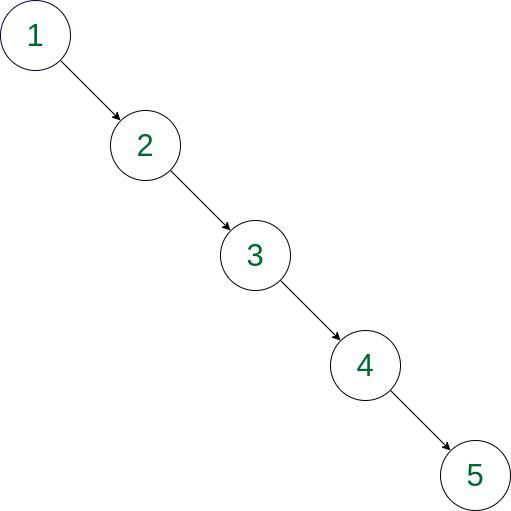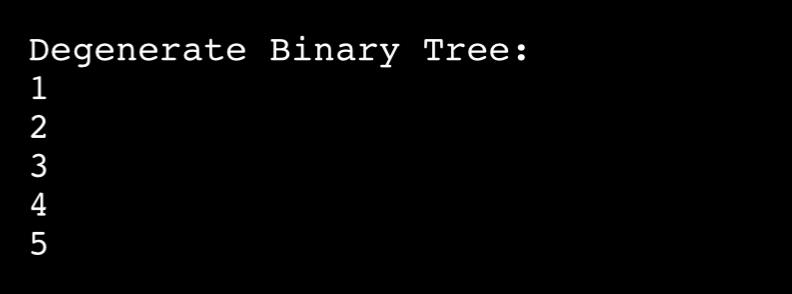GFG App
Open AppBrowser
Continue

# Introduction to Degenerate Binary Tree

Every non-leaf node has just one child in a binary tree known as a Degenerate Binary tree. The tree effectively transforms into a linked list as a result, with each node linking to its single child.

• When a straightforward and effective data structure is required, degenerate binary trees, a special case of binary trees, may be employed. For instance, a degenerate binary tree can be used to create a stack data structure since each node simply needs to store a reference to the next node.
• Degenerate binary trees are less effective than balanced binary trees at searching, inserting, and deleting data, though. This is due to the fact that degenerate binary trees may become unbalanced, resulting in performance that is comparable to a linked list in the worst case.
• Degenerate binary trees are rarely employed by themselves as a data structure in general. Rather, they are frequently utilized as a component of other data structures like linked lists, stacks, and queues. Degenerate binary trees can also be utilized as a particular instance to take into account in algorithms that traverse or search over binary trees.

Degenerate Binary tree is of two types:

• Left-skewed Tree:  If all the nodes in the degenerate tree have only a left child.
• Right-skewed Tree: If all the nodes in the degenerate tree have only a right child.

Examples:

Input: 1, 2, 3, 4, 5
Output:Representation of right skewed tree

Explanation: Each parent node in this tree only has one child node. In essence, the tree is a linear chain of nodes.

Approach: To solve the problem follow the below idea:

Idea is to use handle degenerate binary trees relies on the issue at hand. Degenerate trees may typically be transformed into linked lists or arrays, which can make some operations easier.

Steps involved in the implementation of code:

• Initializing structure of Tree.
• Inserting all data on the right side of a current node.
• Printing node by considering only the right node of each node.

Below is the implementation of the Right-skewed Tree:

## C++

 `// C++ implementation of above approach` `#include ` `using` `namespace` `std;`   `class` `Node {` `public``:` `    ``int` `data;` `    ``Node* left;` `    ``Node* right;`   `    ``Node(``int` `val)` `    ``{` `        ``data = val;` `        ``left = NULL;` `        ``right = NULL;` `    ``}` `};`   `// Function to insert nodes on the right side of` `// current node` `Node* insert(Node* root, ``int` `data)` `{` `    ``if` `(root == NULL) {` `        ``root = ``new` `Node(data);` `    ``}` `    ``else` `{` `        ``root->right = insert(root->right, data);` `    ``}` `    ``return` `root;` `}`   `// Function to print tree` `void` `printTree(Node* node)` `{` `    ``if` `(node != NULL) {` `        ``cout << node->data << endl;` `        ``printTree(node->right);` `    ``}` `}`   `// Driver code` `int` `main()` `{` `    ``Node* root = NULL;` `    ``root = insert(root, 1);` `    ``insert(root, 2);` `    ``insert(root, 3);` `    ``insert(root, 4);` `    ``insert(root, 5);`   `    ``// Function call` `    ``printTree(root);`   `    ``return` `0;` `}`   `// This code is contributed by Prajwal Kandekar`

## Java

 `class` `Node {` `    ``int` `data;` `    ``Node left;` `    ``Node right;`   `    ``Node(``int` `val)` `    ``{` `        ``data = val;` `        ``left = ``null``;` `        ``right = ``null``;` `    ``}` `}`   `public` `class` `Main {` `    ``// Function to insert nodes on the right side of` `    ``// current node` `    ``public` `static` `Node insert(Node root, ``int` `data)` `    ``{` `        ``if` `(root == ``null``) {` `            ``root = ``new` `Node(data);` `        ``}` `        ``else` `{` `            ``root.right = insert(root.right, data);` `        ``}` `        ``return` `root;` `    ``}`   `    ``// Function to print tree` `    ``public` `static` `void` `printTree(Node node)` `    ``{` `        ``if` `(node != ``null``) {` `            ``System.out.println(node.data);` `            ``printTree(node.right);` `        ``}` `    ``}`   `    ``// Driver code` `    ``public` `static` `void` `main(String[] args)` `    ``{` `        ``Node root = ``null``;` `        ``root = insert(root, ``1``);` `        ``insert(root, ``2``);` `        ``insert(root, ``3``);` `        ``insert(root, ``4``);` `        ``insert(root, ``5``);`   `        ``// Function call` `        ``printTree(root);` `    ``}` `}`

## Python3

 `# Python implementation of above approach` `class` `Node:` `    ``def` `__init__(``self``, data):` `        ``self``.left ``=` `None` `        ``self``.right ``=` `None` `        ``self``.data ``=` `data`   `# Function to insert nodes on the right side of` `# current node`     `def` `insert(root, data):` `    ``if` `root ``is` `None``:` `        ``root ``=` `Node(data)` `    ``else``:` `        ``root.right ``=` `insert(root.right, data)` `    ``return` `root`   `# Function to print tree`     `def` `printTree(node):` `    ``if` `node ``is` `not` `None``:` `        ``print``(node.data)` `        ``printTree(node.right)`     `# Driver code` `root ``=` `None` `root ``=` `insert(root, ``1``)` `insert(root, ``2``)` `insert(root, ``3``)` `insert(root, ``4``)` `insert(root, ``5``)`   `# Function call` `printTree(root)`

## C#

 `// C# implementation of above approach` `using` `System;`   `class` `Node {` `    ``public` `int` `data;` `    ``public` `Node left;` `    ``public` `Node right;`   `    ``public` `Node(``int` `val)` `    ``{` `        ``data = val;` `        ``left = ``null``;` `        ``right = ``null``;` `    ``}` `}`   `// Function to insert nodes on the right side of` `// current node` `class` `Program {` `    ``static` `Node Insert(Node root, ``int` `data)` `    ``{` `        ``if` `(root == ``null``) {` `            ``root = ``new` `Node(data);` `        ``}` `        ``else` `{` `            ``root.right = Insert(root.right, data);` `        ``}` `        ``return` `root;` `    ``}` `    ``// Function to print tree` `    ``static` `void` `PrintTree(Node node)` `    ``{` `        ``if` `(node != ``null``) {` `            ``Console.WriteLine(node.data);` `            ``PrintTree(node.right);` `        ``}` `    ``}` `    ``// Driver code` `    ``static` `void` `Main(``string``[] args)` `    ``{` `        ``Node root = ``null``;` `        ``root = Insert(root, 1);` `        ``Insert(root, 2);` `        ``Insert(root, 3);` `        ``Insert(root, 4);` `        ``Insert(root, 5);`   `        ``// Function call` `        ``PrintTree(root);`   `        ``Console.ReadKey();` `    ``}` `}`

## Javascript

 `class Node {` `  ``constructor(val) {` `    ``this``.data = val;` `    ``this``.left = ``null``;` `    ``this``.right = ``null``;` `  ``}` `}`   `// Function to insert nodes on the right side of` `// current node` `function` `insert(root, data) {` `  ``if` `(root === ``null``) {` `    ``root = ``new` `Node(data);` `  ``} ``else` `{` `    ``root.right = insert(root.right, data);` `  ``}` `  ``return` `root;` `}`   `// Function to print tree` `function` `printTree(node) {` `  ``if` `(node !== ``null``) {` `    ``console.log(node.data);` `    ``printTree(node.right);` `  ``}` `}`   `// Driver code` `let root = ``null``;` `root = insert(root, 1);` `insert(root, 2);` `insert(root, 3);` `insert(root, 4);` `insert(root, 5);`   `// Function call` `printTree(root);`

Output

```1
2
3
4
5```

Output :Below is the implementation of the Left-skewed Tree:

## Python3

 `# Python implementation of above approach` `class` `Node:` `    ``def` `__init__(``self``, data):` `        ``self``.left ``=` `None` `        ``self``.right ``=` `None` `        ``self``.data ``=` `data`   `# Function to insert nodes on the left side of` `# current node`     `def` `insert(root, data):` `    ``if` `root ``is` `None``:` `        ``root ``=` `Node(data)` `    ``else``:` `        ``root.left ``=` `insert(root.left, data)` `    ``return` `root`   `# Function to print tree`     `def` `printTree(node):` `    ``if` `node ``is` `not` `None``:` `        ``print``(node.data)` `        ``printTree(node.left)`     `# Driver code` `root ``=` `None` `root ``=` `insert(root, ``1``)` `insert(root, ``2``)` `insert(root, ``3``)` `insert(root, ``4``)` `insert(root, ``5``)`   `# Function call` `printTree(root)`

Output

```1
2
3
4
5```

Time Complexity: O(N)
Auxiliary Space: O(N)

My Personal Notes arrow_drop_up